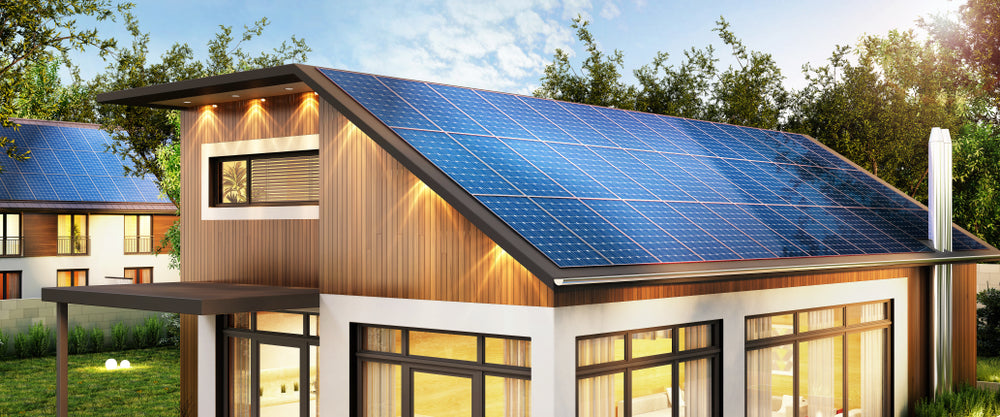# How many solar panels do you need ?To calculate how many solar panels you need, you simply need to do the following:

1. Knowing how much energy your household uses daily?
2. Knowing the climate conditions and peak sunlight hours in your area.
3. Then, divide your household’s hourly energy requirement by the peak sunlight hours to get the amount of energy your panels need to produce daily.
4. Lastly, divide the result by a panel’s wattage to get your target number of panels

How many watts do you currently use?

To determine your home’s average energy requirements look at past electric bills.. Look for “Kilowatt Hours (or kWh) Used” or something similar, and then note the time period represented (usually one month).

You want daily usage for our calculations, though, so if your bill doesn’t show a daily average, just divide the monthly or annual average by 30 or 365 days. Your answer will be in kilowatt-hours (kWh). (And just in case you are wondering, a kilowatt-hour is how much power you are using at any given time multiplied by the total time the power is being used.)

A small home in a temperate climate might use something like 200 kWh per month, and a larger home where air conditioners account for the largest portion of home energy usage might use 2,000 kWh or more. The average U.S. home uses about 900 kWh per month. So that’s 30 kWh per day.

Your average daily energy usage is your target daily average for to calculate your solar needs. That’s the number of kilowatt-hours you need your solar system to produce if you want to cover 100 percent of your energy needs.

Now multiply the average daily usage by 1,000 to convert it to Watts. Divide your average hourly wattage requirement by the number of daily peak sunlight hours for your area this gives you the amount of energy your panels need.

Example :

For an average U.S. home (900 kWh/month) in an area that gets five peak sunlight hours per day and using a panel capacity of 300 Watt

Hourly daily energy requirement = 900/30 = 30 kWh

Amount of energy your panels need to produce every day = 30 / 5 = 6 x 1000 (conversion to Watt) = 6000 Watt to be produced.

=> So your system needs 2 panels to produce